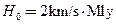Chapter 34, Problem 8PE

Chapter
Section
Textbook Problem

(a) Calculate The approximate age of the universe from the average value of the Hubble constant,To do this, calculate the time it would take to travel 1 Mly at a constant expansion rate of 20 km/s. (b) If deceleration is taken into account, would the actual age of the universe be greater or less than that found here? Explain.

To determine

(a)

The approximate age of the universe from average value of Hubble constant.

Explanation

Given data:

Distance to the galaxy is d=1Mlyor1Mly×106ly1Mly×9.46×1012km1ly.

Hubble constant is H0=20kms/Mly.

Formula used:

The formula for time is,

t=dv   ......... (1)

Calculation:

Substitute the given value in equation (1).

t=1Mly( 10 6 ly 1Mly )( 9.46× 10 12 km 1ly )20km/s=4

To determine

(b)

Wether actual age of the universe be greter or less than found here.

Still sussing out bartleby?

Check out a sample textbook solution.

See a sample solution

The Solution to Your Study Problems

Bartleby provides explanations to thousands of textbook problems written by our experts, many with advanced degrees!

Get Started